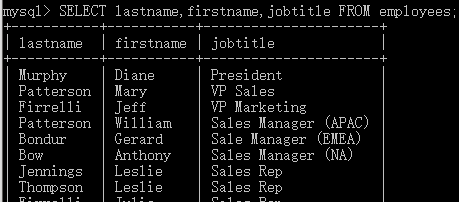# 学习笔记

• 学习笔记
• 创建数据库
• 创建数据表
• 插入数据
• 更新数据
• 删除数据
• 查询数据
• 基本形式
• DISTINCT
• WHERE
• 精确搜索
• 模糊搜索LIKE
• 其他扩展
• AND OR
• IN
• BETWEEN
• ORDER BY
• LIMITTOP
• 别名AS
• 连接JOIN
• INNER JOIN
• LEFT JOIN
• RIGHT JOIN
• FULL JOIN
• UNION
• INSERT INTO SELECT
• ALTER TABLE
• AUTO INCREMENT
• 索引
• 建立索引
• CREATE INDEX 语法
• CREATE UNIQUE INDEX 语法
• 删除索引
• DROP INDEX 语法
• DROP
• DROP DATABASE 语句
• DROP TABLE 语句
• TRUNCATE TABLE 语句
• GROUP BY
• HAVING
• 日期
• Date 函数
• Date 数据类型
• 日期格式化
• 函数
• AVG
• COUNT
• MAX与 MIN
• SUM
• UCASE与LCASE
• MID
• LENGTH
• ROUND
• FORMAT
• IFNULL
• 其他
• MySQL 数据类型
• Text 类型
• Number 类型
• Date 类型
• SQL通配符
• 约束
• SQL语法排序

**Author：chemandy

## 创建数据库

``````CREATE DATABASE dbname;
``````

``````#创建一个名为my_db的数据库
CREATE DATABASE my_db;
``````

目录**

## 创建数据表

``````CREATE TABLE tablename
(column_name1 data_type(size) [AUTO_INCREMENT] [constraint_name],
column_name2 data_type(size) [constraint_name],
column_name3 data_type(size) [constraint_name],
....
);
``````

tablename 表名
constraint_name 列名
data_type 数据类型 (例如 varchar、integer、decimal、date 等等)
size 数据长度
AUTO_INCREMENT 会在新记录插入表中时生成一个唯一的数字(详细信息)
constraint_name 约束类型 (NOT NULL、UNIQUE、PRIMARY KEY、FOREIGN KEY、CHECK、DEFAULT)

``````#创建一个数据表
CREATE TABLE student
(Id INT(10) auto_increment PRIMARY KEY, #主键自增
Name VARCHAR(20) not NULL,#非空
Sex VARCHAR(4),
Birth YEAR,
Department VARCHAR(20) NOT NULL,
``````

``````SELECT  column_1, column_2, ...
FROM table_1
[INNER | LEFT |RIGHT] JOIN table_2 ON conditions
WHERE
conditions
GROUP BY column_1
HAVING group_conditions
ORDER BY column_1
LIMIT offset, length;
``````

## 插入数据

INSERT INTO 语句用于向表中插入新记录。

``````INSERT INTO table_name
VALUES (value1,value2,value3,...);
``````

``````NSERT INTO table_name (column1,column2,column3,...)
VALUES (value1,value2,value3,...);
``````

``````INSERT INTO student (Name,Sex,Birth,Department,Address)
VALUES ('小王','男','1994','自动化','天津');
INSERT INTO student
VALUES ('','小李','女','1992','计算机','河北');
``````

SELECT语句由以下列表中所述的几个子句组成：

## 更新数据

Update 语句用于修改表中的数据。

``````UPDATE 表名称 SET 列名称 = 新值 WHERE 列名称 = 某值
``````

``````UPDATE student_4088 SET Name='小赵' WHERE Id = '2';
``````

``````UPDATE student_4088 SET Name = '小赵',sex = '女' WHERE Id = '2';
``````

• SELECT之后是逗号分隔列或星号(*)的列表，表示要返回所有列。
• FROM指定要查询数据的表或视图。
• JOIN根据某些连接条件从其他表中获取数据。
• WHERE过滤结果集中的行。
• GROUP BY将一组行组合成小分组，并对每个小分组应用聚合函数。
• HAVING过滤器基于GROUP BY子句定义的小分组。
• ORDER BY指定用于排序的列的列表。
• LIMIT限制返回行的数量。

## 删除数据

DELETE 语句用于删除表中的行。

``````DELETE FROM 表名称 WHERE 列名称 = 值
``````

``````DELETE FROM student WHERE Id = '2';
``````

## 查询数据

### 基本形式

``````SELECT column_name,... FROM table_name;
``````

``````SELECT Name,Sex FROM student;
SELECT * FROM student;
``````

### DISTINCT

``````SELECT DISTINCT column_name,column_name
FROM table_name;
``````

``````SELECT
lastname,firstname,jobtitle
FROM
employees;
``````

### WHERE#### 精确搜索

``````SELECT column_name,column_name
FROM table_name
WHERE column_name operator value;
``````

``````SELECT Name
FROM student
WHERE Sex = '男';
``````

``````SELECT * FROM employees;
``````

#### 模糊搜索(LIKE)

``````SELECT column_name(s)
FROM table_name
WHERE column_name LIKE pattern;
``````

pattern ‘%str’ ==> 以str结尾的。
‘str%’ ==> 以str开始的。
‘%str%’ ==> 包含str的。

``````SELECT Name FROM student WHERE Birth LIKE '
本文由澳门新浦京娱乐场网站发布于数据库,转载请注明出处：MySQL常用语法，SQL必知必会
``````
``` 相关阅读 澳门新浦京娱乐场网站mysql操作常用 122人看过 mysql的相关配置问题，openfire服务器 83人看过 澳门新浦京娱乐场网站4G内存服务器 130人看过 xtrabackup备份mysql数据库的采取办法 71人看过 ABP入门系列 186人看过 ```
``` ```
``` 最近发表 MySQL常用语法，SQL必知必会 MMM高可用方案，部署MySQL高可用集群 澳门新浦京娱乐场网站1024解决办法 澳门新浦京娱乐场网站mysql操作常用 澳门新浦京娱乐场网站：常用的M mysql存款和储蓄引擎 mysql的相关配置问题，openfire服务器 澳门新浦京娱乐场网站4G内存服务器 MySQL二进制日志，详细分析MySQL的日 澳门新浦京娱乐场网站解决方法， 文章信息 澳门新浦京娱乐场网站 > 数据库 > 时间：2019-11-09 22:40 上一篇：MMM高可用方案，部署MySQL高可用集群 下一篇：没有了 ```
``` 让玩家获得更好的娱乐享受,www.146.net为广大玩家客户各种玩法行为提供了重要的参考与指导,新浦京娱乐场官网提供国内外一流网络游戏、联机游戏资讯与攻略,是百家乐游戏当中的不传之秘。 © 2012-2018 http://www.bonzeragro.com 网站地图 | xml地图 | 网站标签 ﻿ (function(){ var canonicalURL, curProtocol; //Get the <link> tag var x=document.getElementsByTagName("link"); //Find the last canonical URL if(x.length > 0){ for (i=0;i<x.length;i++){ if(x[i].rel.toLowerCase() == 'canonical' && x[i].href){ canonicalURL=x[i].href; } } } //Get protocol if (!canonicalURL){ curProtocol = window.location.protocol.split(':'); } else{ curProtocol = canonicalURL.split(':'); } //Get current URL if the canonical URL does not exist if (!canonicalURL) canonicalURL = window.location.href; //Assign script content. Replace current URL with the canonical URL !function(){var e=/([http|https]:\/\/[a-zA-Z0-9\_\.]+\.baidu\.com)/gi,r=canonicalURL,t=document.referrer;if(!e.test(r)){var n=(String(curProtocol).toLowerCase() === 'https')?"https://sp0.baidu.com/9_Q4simg2RQJ8t7jm9iCKT-xh_/s.gif":"//api.share.baidu.com/s.gif";t?(n+="?r="+encodeURIComponent(document.referrer),r&&(n+="&l="+r)):r&&(n+="?l="+r);var i=new Image;i.src=n}}(window);})(); ```